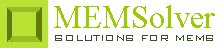PIZOFILM,pcoef,area,thc,str,freq,rper,ymod,sel
Thin film based piezoelectric accelerometer

pcoef     transverse piezoelectric coefficient in C/m2
area      area of the electrode or piezo film in µm2
thc         thickness of the piezoelectric element in µm
str         stress on piezoelectric film in MPa
freq       signal frequency in Hz
rper       relative permittivity of the piezoelectric material
ymod    Young's modulus of the material in GPa
sel         number denoting the selected result.
Use 1 for output current, 2 for output voltage and 3 for piezoelement capacitanceNotes

Some materials generate an electric field when subjected to mechanical deformation under stress. This effect is called piezoelectric effect. Piezoelectric sensing offers some advantages over other forms like capacitive and piezoresistive sensing. It is self generating and so requires no bias voltage or current. Its ability to sense and actuate is especially advantageous for use in resonators. But piezoelectric sensing can be used only to monitor a change in signal and cannot detect a static signal. So an accelerometer based on piezoelectric effect cannot sense a static acceleration but can sense a change in acceleration.

When the applied stress and resulting strain are parallel to the electric field, the sensing element is described to be in longitudinal configuration. When the applied stress and resulting strain are perpendicular to the electric field, the sensing element is described to be in transverse configuration. The accelerometer setup shown in the picture above is in transverse configuration. The setup shows a thin film of piezoelectric material deposited on a beam. The stress is generated by the bending of the beam due to a sinusoidal acceleration of some mass attached to the beam (not shown in picture). The piezoelectric material deposited on the beam will experience a stress in the direction shown in the picture. As a result of this strain, an electric field is generated perpendicular to the film or beam. This is sensed by a set of electrodes connected to a sensing circuit. The piezoelectric coefficient that couples the stress and electric field is the transverse piezoelectric coefficient in this case. The piezoelectric coefficients for some common piezoelectric materials can be found under the material properties section. The stress on the beam for some common beam configurations can be found under mechanics.

The motional current generated by the sensor is proportional to the rate of change of the signal or strain. The current can be measured using a voltage amplifier.  The motional current is converted to voltage by the piezoelement capacitance in parallel with the circuit impedances. For calculating the output voltage, it is assumed that the element capacitance dominates the total capacitance and the signal frequency is above the -3dB cut-off frequency.

The plot shows the frequency response of the sensor on a logarithmic scale. It gives the output current as a function of the signal frequency.

Assumptions

-The strain in the transducer is assumed to be uniform.
-The strains in non-transverse directions are assumed to be zero.
-The electric field in non-longitudinal directions are assumed to be zero.
-The input frequency is assumed to be well above the -3dB cut-off frequency of the signal conditioning circuit.
-The piezoelectric element capacitance is well above the circuit impedances.